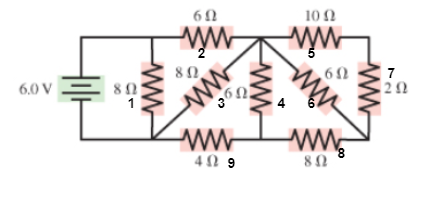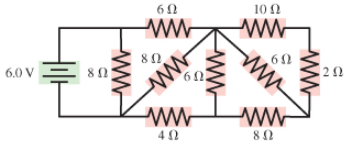# Problem: Consider the circuit shown in (Figure 1).What is the current through the battery? Express your answer to two significant figures and include the appropriate units.

🤓 Based on our data, we think this question is relevant for Professor Younes' class at GWU.

###### FREE Expert Solution

Current:

$\overline{){\mathbf{i}}{\mathbf{=}}\frac{\mathbf{V}}{{\mathbf{R}}_{\mathbf{e}\mathbf{q}}}}$

Equivalent resistance for resistors in series:

$\overline{){{\mathbf{R}}}_{\mathbf{e}\mathbf{q}}{\mathbf{=}}{{\mathbf{R}}}_{{\mathbf{1}}}{\mathbf{+}}{{\mathbf{R}}}_{{\mathbf{2}}}{\mathbf{+}}{{\mathbf{R}}}_{{\mathbf{3}}}{\mathbf{+}}{\mathbf{.}}{\mathbf{.}}{\mathbf{.}}{\mathbf{+}}{{\mathbf{R}}}_{{\mathbf{n}}}}$

Equivalent resistance for resistors in parallel:

$\overline{)\frac{\mathbf{1}}{{\mathbf{R}}_{\mathbf{e}\mathbf{q}}}{\mathbf{=}}\frac{\mathbf{1}}{{\mathbf{R}}_{\mathbf{1}}}{\mathbf{+}}\frac{\mathbf{1}}{{\mathbf{R}}_{\mathbf{2}}}{\mathbf{+}}{\mathbf{.}}{\mathbf{.}}{\mathbf{.}}{\mathbf{+}}\frac{\mathbf{1}}{{\mathbf{R}}_{\mathbf{n}}}}$

Label the resistors as follows:Resistors 5 and 7 are in series.

R57 = 10 + 2 = 12Ω

R57 is in parallel with R6

R567 = (12)(6)/(12 + 6) = 4Ω

R567 is in series with R8

R5678 = 4 + 8 = 12Ω###### Problem Details

Consider the circuit shown in (Figure 1).

What is the current through the battery? Express your answer to two significant figures and include the appropriate units.